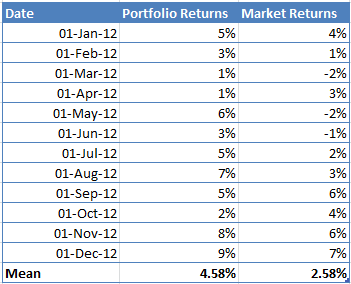# Jensen’s Alpha Calculator in Excel

The Jensen’s Alpha is a popular risk-adjusted performance measure used by portfolio managers to determine how much excess returns their portfolio has generated over and above the market returns as suggested by the CAPM model.

A positive alpha indicates that the portfolio has outperformed the market, and vice versa.

The Jensen’s Alpha can be calculated using the following formula:

$\alpha =R_{P}-\left ( R_{f}+\beta \left ( R_{M} -R_{f}\right ) \right )$

Where:

• Rp = Returns of the Portfolio
• Rf = Risk-free rate
• β = Stock’s beta
• Rm = Market return

Let’s look at how Jensen’s Alpha can be calculated in Excel.

Step 1: Let’s say we have the following returns data for our portfolio and a benchmark index in excel. The first thing we need to do is calculate the mean of both the returns.Step 2: Once we have the data, we need to define a risk-free rate. Let’s say the risk-free rate is 1.5%.

Step 3: The next step is to calculate the portfolio Beta, which will be used to calculate the expected returns using CAPM. Beta will be calculated using the following formula:

β = Covariance(Rp,Rm)/Variance(Rp)

Use the formula COVARIANCE.P(), and VAR.P() in excel to perform the above calculations.In our example, the value of Beta is 0.61.

Step 4: Now that we have the Beta, we can calculate the expected return using CAPM.

E(Rp) = 1.5%+0.61*(2.58%-1.5%)

E(Rp) =2.16%

Step 5: The last step is to calculate Jensen’s Alpha by subtracting the expected returns from the actual mean portfolio returns.

Jensen’s Alpha = 4.58% - 2.16% = 2.42%